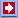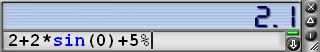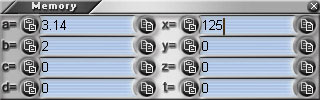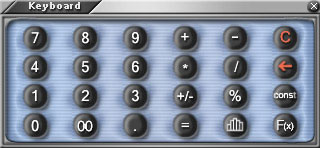Software Online Library ContactsSoftware: Business&GamesCalculator Download Analyzer Mail NavigatorOnline: Stat Calc Y(X) Finder 2D Plotter 3D Plotter Science CalcLibrary: Data Mining Statistics Machine Learning Genetic Algorithms Neuronets Fuzzy logic ForecastLinksContacts## Help on Using Business&Games Calculator

http://qudata.com/calculator/

## Contents

 Calculator in a Nutshell Detailed Instrustions Advanced Panels Diversions

## Why Business&Games Calculator Is Worth Using?

Business&Games Calculator combines exclusive convenience in work, nice interface and functional capacity. Its basic features are:

• Form that can be easily adjusted. You can work either only with the narrow strip, placed over the other applications, or you can activate different Calculator panels and place them at your discretion.
• You can enter numbers and functions not only with the graphic 'keyboard' pad, but also from the PC keyboard using the Calculator line of input. You will be able to see the expression that is calculated, not just the result.
• Working with the memory in B&G Calculator is very convenient. One can use a set of cells (variables) for storage of intermediate results. You can save a variable to or load it from the memory by a single mouse click or pressing the symbol '=', for example, x=7+2. You can see the current values of all memory cells on the panel Memory.
• The list of calculation history allows you to return to the earlier calculated expressions. The history can be printed or saved in the HTML file.
• High-capacity set of mathematical, statistical, special and financial functions allows you to carry out diversified calculations. B&G Calculator contains a number of panels for statistical computation, graphs plotting, financial computations and many other things. Working with percents and conversion system between different number systems is very convenient.
• B&G Calculator also functions as the reference book on different mathematical and physical constants. You can always convert pounds into kilos, insert the distance between the Earth and the Sun into your calculations and enter your own constants easily and without any problem.
• Skins allow you to change the Calculator's appearance. For each skin you can choose font size and type. It is also possible to set the mode of random skin, and then Calculator will greet you every day with new design.
• In case you are tired and need a rest, you can play the most enthralling games Bubble Shooter and Gem Slider that are strongly recommended by psychologists as the best way to relax. Bubble Shooter is placed in one of the Calculator panels.

It is not necessary to read all this help to start working with Calculator. The following part will be enough for you to make the work more comfortable.

## Master it in 5 minutes!

Business&Games Calculator may be launched from the system tray (at the bottom right corner of the desktop).

The main window - consisting of the result indicator, the line of expression input, buttons 'close', 'minimize', 'menu' - is always shown in B&G Calculator no matter what regime do you use.The main window allows you to carry out the most of operations with Calculator. For that (if calculator is in focus) you have to begin typing the expression you want to calculate. Examples of expressions:

 ``` 137 + 3.14 (2 + 3) * 5 + 2^5 10 + 20.5 + 10% 25! - sin(3) + erf(gamma(pi)) ```

When you type the expression, Calculator tries to show the result at once. If the expression is typed correctly, the indicator (on the right of line of input) is green, otherwise red.

Typing expressions, you may use Latin letters x, y, z,.. as memory cells. To save the expression result in the memory cell x, you should type 'x = expression'. Later on memory cell x can be used as follows:

 ` x = 128 / 5 3 * x + 2 z = x + y^2 `

Besides the memory cells you can use mathematical constants pi=3.1415..,, e=2.71828. In expression you can also use operations with percents calculation. Along with the decimal numbers, the hex ( 0xff01a ) and binary ( 0b11001 ) numbers, as well as numerous mathematical functions can be entered. By default the result is in decimal system. If you put the symbol '#' at the beginning of the input line, indicator will show the result in hex system, and if you put symbol '@' in binary system. More detailed information concerning the expression syntax and the list of available functions can be found in the part Mathematical Expressions.If after the input of expression you press Enter, it will be marked and placed to the history list. The input line is cleared when typing the next expression. Line of input is supplied with the button for getting the list of earlier entered expressions.

The main window size can be changed with the help of mouse, dragging the right bottom corner. Changing size allows increasing or decreasing the number of result's significant digits.

Calculator Menu is called by clicking the button Menu or with right mouse click anywhere on the Calculator. From menu you can activate other panels. For example, click menu button and check the item 'Memory'. You will see the panel with the list of memory cells' values. You can separate this panel (dragging its title with the mouse) and move it to the place you want. It can be moved and placed on the right from the main window. If the distance between the panels is not large, they stick to each other and form one window. A double mouse click on the window title will minimize it to a narrow strip that can be restored later. Button 'X' in the top right corner of each panel closes it (not exiting the Calculator). To close Calculator itself, just click the button 'X' on the main panel!

Opened Memory panel allows you to see and, if you want, edit the memory cells' values. The buttons (those on the right and left of the cell) correspondingly save the current value to the memory or place the cell's value in the expression line.

If you got used to using the standard Calculator keyboard, activate the panel 'keyboard' through the main menu. To get additional menu with the list of available constants, press Const button, menu with the list of functions - button F(x).Calculator menu has a number of useful option setting. In particular, very convenient is 'Always top' regime, when the Calculator is always above the other windows. Click 'Minimize' button of the main window if some additional panels are opened and Calculator panel (being at hand) will not bother you.

## Work with Percentages

In Business&Games Calculator a very convenient system for working with percents is realized. For example, the expression

 ` 53 + 22 + 10% `

means that we, at first, sum up two numbers, and then add 10 percents to the result. If it's necessary to add percent not to all the expression but to a part of it, you should take this part and percent in parentheses:

 ``` 53 + (22 + 10%) ```

Now we add 10 percents to number 22, then add the result to 53 and we get 55.2, whereas in the previous example we get 7.5 (10 percents from 75=53+22). Operations on percent subtraction are carried out similarly.

If we multiply the number by percent, that results in taking percent from the given number:

 ``` 20 * 10% 50 + 20 * 10% (50 + 20) * 10% ```

The result of the first example will be 2 (10 percents from 20). In the second one, we first take 10 percents from 20, and then add 50 to the result (multiplication has higher priority than addition or subtraction). If it's necessary to take the percent from the whole expression, it should be taken in the parentheses as in the third example, where the result will be equal to 7 (10 percents from 70=50+20), in contrast to the second line, the result of which is 52=50+2.

As the percent division is used extremely seldom in the desktop Calculators, here it has a meaning different from the common one. When dividing the number by the percent, we 'take' the number from the percent. In other words, percent division is inverse to percent addition. For example, we know that the cost of goods with a 30 percent margin is 65. To get the cost of goods without the margin you should do:

 ``` 65 / 30% ```

We will get 50 as a result. Indeed, 50+30% = 65. Note that for operations with percents addition and subtraction are not reverse as they are taken from different quantities. ( 50 + 30 % ) - 30% doesn't equal 50, whereas ( 50 + 30 % ) / 30% will equal 50.

Expression that contains percents one after another is calculated by applying the 'taking the percent'; operation. For example, if you have to add 20 percent to the cost of goods of 50, and then add 10 percent to result, you should type:

 ``` 50 + 20% + 10% ```

You will receive 66 = (50 + 20 %) + 10% = 60 + 10%, not 65 as it would be when adding 30 percents.

## Mathematical Expressions

Business&Games Calculator makes calculations with integer numbers (4, 137, -12) or real numbers (0.1, 123.4, 12e-4). Notation 12e-4 means 12 *10-4. Besides, hex numbers can be used in expressions. For that you should put prefix '0x' before the hex number: 0xFF is 256, and 0x000a is 10. Binary numbers are formed in the same way, with prefix '0b': 0b1001 is 9.

Numbers are involved in usual mathematical operations +, -, *, /, as well as in those of rising to a power ^ (2^3 equals 8). Expression can be grouped with parentheses ( , ). The sign ! can be placed after the number or variable, that means taking factorial of ( x!=1*2*..*x )

In expression you can use mathematical constants pi=3.1415.., e=2.71828 and variables x,y,z,t,a,b,c,d. The names of variables are predetermined: you are allowed to use only those present in the 'Memory' panel. To remember the result into the memory cell, it should be assigned by means of the equal sign, after that the name of this variable can be used in expressions. After the assignment, the cell x contains the value of the expression, that goes after equal sign "=" (in panel 'Memory'). New assignment or editing on the «Memory» panel can change cell's value. It is possible to assign a value to a variable along with using it in the expression. For example, when you enter 2+(x=5+7)*4 the first action will be saving the value 5+7 into the cell x, then the calculation of expression 2+(5+7)*4

Variable v always stores the last result. That is why, having entered some expression, you can use its value in the following:

 ``` 32 + 748 / 67 v + 100 v + 300 ```

Note, that the value of the variable v is changed when each new result is calculated. That is why we do not recommend using it as a long-term storage of the user's values.

The case of the letters in expressions does not matter. sin(X) and SiN(x) are the same.

## Mathematical Functions

The following basic mathematical functions can be used in expressions

• sqrt(x) - square root. Same as x^0.5
• sqr(x) - Square of the number. Same as x^2
• exp(x) - Number "e=2.71828.." raised to a power x. Equivalent e^x
• ln(x) - natural logarithm (to the base of *e*) ( ln( exp(x) ) equals x )
• log(x) - logarithm to the base of 10 ( log( 10^x ) equals x )
• log2(x) - logarithm to the base of 2 ( log2( 2^x ) equals x )
• log(x,base) - logarithm x to the base of *base*

Standard trigonometrical functions:

• sin(x) - sine
• cos(x) - cosine
• tg(x) - sin(x)/cos(x)
• ctg(x) - cos(x)/sin(x)

Inverse trigonometrical functions:

• asin(x) - arc sine (asin (sin(x)) equals x)
• acos(x) - arc cosine (acos (cos(x)) equals x)
• atg(x) - arc tangent (atg (tg(x)) equals x)
• atg2(x,y) - arc tangent (x,y must be between pi and -pi)
• actg(x) - arc cotangent (actg (ctg(x)) equals x)

Their hyperbolic analogues:

• sh(x) - hyperbolic sine equals (exp(x)-exp(-x))/2
• ch(x) - hyperbolic cosine equals (exp(x)+exp(-x))/2
• th(x) - hyperbolic tangent equals sh(x)/ch(x)
• cth(x) - hyperbolic cotangent equals ch(x)/sh(x)

By default, trigonometrical functions make calculations in radians. The following functions convert degrees into radians and vice versa.

• deg(x) - conversion of x radians into degrees

Factorial functions:

• fact(x) - factorial of the number x fact(x) = 1*2*3*..*x
• gamma(x) - Euler's gamma function fact(x)=gamma(x+1)
• gammaln(x) - natural logarithm of gamma function ln(gamma(x))
• beta(x,y) - beta function, that is gamma(x+y)/(gamma(x)gamma(y))

As factorial function is a very fast growing one, for large values of x an overflow can occur. In this case it is often better to use logarithmic gamma function gammaln. Note, that all factorial functions also work with real values of argument.

Statistical functions:

• erf(x) - error function
• gammap(x, p) - incomplete gamma function
• chisqr(x) - chi-square
• tstudent(x,y) - Student function
• betai(x,n,m) - incomplete beta function

Inverse statistical functions:

• ierf(x) - inverse error function
• igammap(x, p) - inverse incomplete gamma function
• ichisqr(x) - inverse chi-square
• itstudent(x,y) - inverse Student function
• ibetai(x,n,m) - inverse incomplete beta-function

Prefix 'i' means inverse. So, for example, ierf(erf(x)) equals x and so on.

Bitwise logical functions:

• not(x) - bit inversion
• or(x,y) - logical bitwise operation 'or'
• and(x,y) - logical bitwise operation 'and'
• xor(x,y) - bitwise operation 'excepting or'
• shr(x,n) - logical shift x to the right on n bits
• shl(x,n) - logical shift x to the left on n bits
• if(x,v1,v2) - if x is not equal to zero, returns v1, otherwise v2
• gt(x,y) - if x > y returns 1, otherwise 0
• ge(x,y) - if x > y or x=y returns 1, otherwise 0
• lt(x,y) - if x< y returns 1, otherwise 0
• le(x,y) - if x < y or x=y returns 1, otherwise 0
• eq(x,y) - if x = y returns 1, otherwise 0
• ne(x,y) - if x = y returns 1, otherwise 0

If the argument of bitwise functions is not integer, it is rounded, and then the operation takes place. Look also the section Work with non-decimal systems of calculation.

The following functions are also allowed:

• abs(x) - absolute value of number x (abs(-3) equals 3)
• power(x,y) - power, equivalent to x^y
• sign(x) - sign of x: 1 if x>0, -1 if x<0, 0 if x = 0.
• floor(x) - the largest integer that is less than x (floor(1.5) equals 1)
• ceil(x) - the smallest integer that is larger than x (ceil(1.2) equals 2)
• round(x) - rounds to the nearest integer (round(1.2) is equal to 1, round(1.5) equals 2)
• mod(x, y) - remainder of the division of x by y (mod(7,3) equals 1)
• rnd() - random number evenly distributed between 0 and 1
• rndg() - normally distributed random number with zero average and unit dispersion

## Work with Non-Decimal Systems of Calculation

Beside the decimal numbers, B&G Calculator understands integer constants in binary and hex notation. They can be entered along with usual numbers. You should only use prefix '0x' before the hex number: 0xFF is 256, and 0x000a is 10. The binary numbers are formed similarly, with the help of prefix '0b': 0b1001 is 9.

By default the calculation result is shown in the decimal system of calculation. There are several ways to see it in hex or binary system. For those who type expression using keyboard, not a mouse, the following way will be ok. Put the symbol '#' at the beginning of expression to see the calculation result in hex system, and '@' in binary system.

It is also possible to change the calculation system through menu. In any case, data output in the system different from decimal one will be indicated in the first field of results with marks 'hex' or 'bin'.

It is worth noting that large hex numbers are stored in the real form with the round-off effect. So the number 0xffffffffffff will appear as 0x1000000000000.

## History Panel. Work with Previous Calculation Results

Panel 'History' may be called from menu, or by clicking the 'history' dropdown button that is on the right of the input line on the main window. After expression calculation (when you press 'INPUT/ENTER' or '=' on the keyboard panel), the expression and the result are placed in the window with history of calculations. You can return to any expression you have worked with before at any moment (fixing it up or typing something new on its base). A double click on the line in the history window puts the expression back to the field of input (on the main window). Separate history lines can be copied to the buffer ('Copy') and deleted (button 'Del'). The history may be cleared ('Clear'). It is possible to form report on the calculations made ('Report'); a file in HTML format with all previous calculations will be saved to disk.

## Convert panel. Conversion of units of measure.

Panel 'Convert' is called from menu. Use this panel to calculate unit conversions. All unit conversion templates function similarly. In the top drop-out list you can choose the kind of units (length, volume, weight, and so on) for which you want transformation be carried out.Then you choose source and target units, enter the value and get result in the other field.

## Statistics Panel for Statistical Calculations

Panel 'Statistics' is called from the main menu or by pressing the corresponding button on the keyboard panel. Even if you are not interested in statistics, it's very convenient to use this panel for summing up the number sequence, typing them or copying from the clipboard, for example. Calculated values (for example, sum) can be used in Calculator as variables with values, correspondent to those in statistics window. Having typed 'sum' in the input field, we receive the sum of all numbers of the window 'Statistics' on the indicator.

You can calculate statistical parameters (sum, mean and so on) with the help of Statistics.

If you enter several numbers, separated by space, in the editing field (placed on statistical Calculator), the following statistical parameters will be calculated:

• num - the number of entered numbers
• sum - their sum
• min - minimum value
• max - maximum value
• range - range equal to max-min
• mean - mean value
• sigma - error (square root of dispersion)
• deviation - mean value of module of deviation from mean
• lquart - left quartile: 25% of numbers are less than this value
• median - median: 50% of numbers are less (greater) than this value
• rquart - right quartile: 25% of numbers are greater than this value
• mode - the value corresponding to the maximum on histogram
• skewness - asymmetry of distribution; it is equal to zero for normal distribution
• excess - excess; it is equal to zero for normal distribution.

When typing the expression on the main panel of Calculator, you can use variables num, sum and others, calculated by statistical Calculator.

Panel Statistics has the following buttons:

• "Calc" - Allows to recalculate statistics values
• "Clear" - Clears the input field
• "Sort" - Sorts the entered numbers
• "Normal" - Generates a set of random numbers normally distributed with zero mean and unit dispersion.

In the center of the panel you can see histogram graph of the series of numbers, as well as a graph of normal distribution with given mean and sigma.

## Bubble Shooter Game

This game with its simplicity and fascination is competitive with Tetris. However it has useful psychological influence. It helps people to get rid of tiredness, to relax. Investigations showed that playing Bubble Shooter makes you mind more stable and plastic.

The rules are rather simple. You should clear the board of colored bubbles, shooting at them with the mouse. You shoot at them with more bubbles, and when three or more of the same color come together, they all explode. If you shoot out all the bubbles of some color, say red, no more red bubbles will appear on the board. This would ease things considerably. It is more interesting and needs great playing skills to keep playing the game with bubbles of 2 or 3 colors as long as possible to get more score. There can be no draw in this game, either you clean all the bubbles off, or they move all the way down on you. No compromise. Indicator shows you the number of attempts left. The board is cleared - you are the winner, the board is filled - you lose.

Bubble Shooter Game is licensed by Absolutist.com. If you wish to use the full version of this game, with skill selection and saving on disk enabled, after the trial period, please download it from http://absolutist.com/bubbles/

## Gem Slider Game

This game is not as simple as it may seem, and quite addictive. This game is real fun to play.

The goal is to remove all the gems from the board, sliding them from the edges. In order to do this you have to slide gems from sides of the board. Click on any gem located somewhere along the border of the board and a new gem will appear close to it, this new gem will begin to slide towards the opposite side of the board. It will slide until it hits the board's border or another gem that has a neighbor gem along the line of movement of the sliding gem. If that gem doesn't have such neighbor the sliding gem will push it until it will hit the border of the board or some gem. If sliding gem joins group of three or more gems of the same type - all these gems disappear. Looks simple, but... Try it yourself and you'll have a lot of fun!

Gem Slider is licensed by Absolutist.com. If you wish to get the full version of the game (full screen, all the levels, save and publishing of results), please please download it from http://absolutist.com/gslider/

A collection of skins for the Calculator can be found at

http://qudata.com/calculator/skins.html